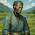Sunday, 2 December 2012

Check if one string is permutation of another string

You have two strings and you need to decide if the first one is a permutation of the other one. The solution uses HashMap data structure where each symbol of the first string is stored as a key and its value is the number of times this symbol appears. Then you loop through the second string and for each character which is a key in the HashMap you decrease the number of times this character appeared in the first string. In the end if the two strings are permutations of each other,  you will end up with a HashMap with all values 0:

```boolean arePermutations(String str1, String str2) {
if (str1.length() != str2.length()) {
return false;
}
HashMap hashMapChars = new HashMap();
// enter the chars and the number of times each of them appears
for (int i = 0; i < str1.length(); i++) {
if (hashMapChars.containsKey(str1.charAt(i))) {
int count = hashMapChars.get(str1.charAt(i));
hashMapChars.put(str1.charAt(i), count + 1);
} else {
hashMapChars.put(str1.charAt(i), 1);   }
}
}
// for each char in str2 decrease the value number
for (int i = 0; i < str2.length(); i++) {
if (hashMapChars.containsKey(str2.charAt(i))) {
int count = hashMapChars.get(str2.charAt(i));
count--;
if (count < 0) {
return false;
}
hashMapChars.put(str2.charAt(i), count);
} else {
return false;
}
}
return true;
}
```

1.Use of an array would be much faster for large strings I think !

2.Or sort them both and compare.

3.sorting them both and comparing works if you are allowed to modify the existing strings. Otherwise this method isn't bad.

4.where is the case where count can be greater than 0 at the end of string 2?

1.We checked in the first line if the strings are of equal length - so if they are, and none of the counts are below zero, then they shall all be zero.

5.This method gives us O(n) time at the cost of the memory size of a hashmap. Sorting would save us the memory cost, but the fastest sorting algorithm would produce O(nlogn) results. Also the cost of comparing after sorting should be taken into account.